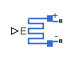# Strain Gauge

Deformation sensor

•Libraries:
Simscape / Electrical / Sensors & Transducers

## Description

The Strain Gauge block represents a sensor that generates a change in resistance as a function of strain using the following equation:

`$\frac{\Delta R}{R}=K\epsilon$`

where:

• ΔR/R is the fractional change in resistance.

• $\epsilon$ is the strain at port E.

• K is the Gauge factor parameter value.

## Ports

### Input

expand all

Physical signal port associated with the strain input.

### Conserving

expand all

Electrical conserving port associated with the strain gauge positive port.

Electrical conserving port associated with the strain gauge negative port.

## Parameters

expand all

Unstressed gauge resistance.

The ratio K of the fractional change in resistance to the fractional change in length.

## Version History

Introduced in R2008a